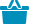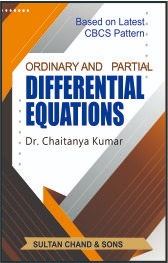Cart

# Ordinary and Partial Differential Equations#### Quick Overview

Authored By

Publisher: Sultan Chand & Sons

Publishing Year: 2021

Size (mm): 220.00 x 160.00

ISBN: 93-5161-197-4

Page nos.: xvi + 320

MRP: 250.00

Subject: Mathematical Sciences

₹250.00

This book has been designed for B.A. (Prog.) III Year, Semester-V, B.Sc. (Hons.) (Mathematics and Physics) and GE-3 (for all honours courses) students of all universities of India following Choice Based Credit System (CBCS). It is also useful for B.Tech students of various universities and various competitive examinations. The students of open and distance education courses will find the book most useful.

• An Introduction to Differential Equations
• Ordinary Differential Equations of Order One and First Degree
• Differential Equations of First Order but Not to First Degree
• The Wronskian and its Properties
• Linear Differential Equation with Constant Coefficients
• Homogeneous Linear Differential Equations
• Method of Variation of Parameters
• Simultaneous Linear Differential Equations
• Total Differential Equations
• Linear Partial Differential Equations – Formation, Solution of First Order Equations
• Non-Linear Partial Differential Equations of Order One
Examination Papers

ISBN13: 978-93-5161-197-4

Weight: 0.00

Edition: 1st Edition 2021

Language: English

Title Code: TC 1238

#### Author

Authored By : Kumar Chaitanya (Dr.)